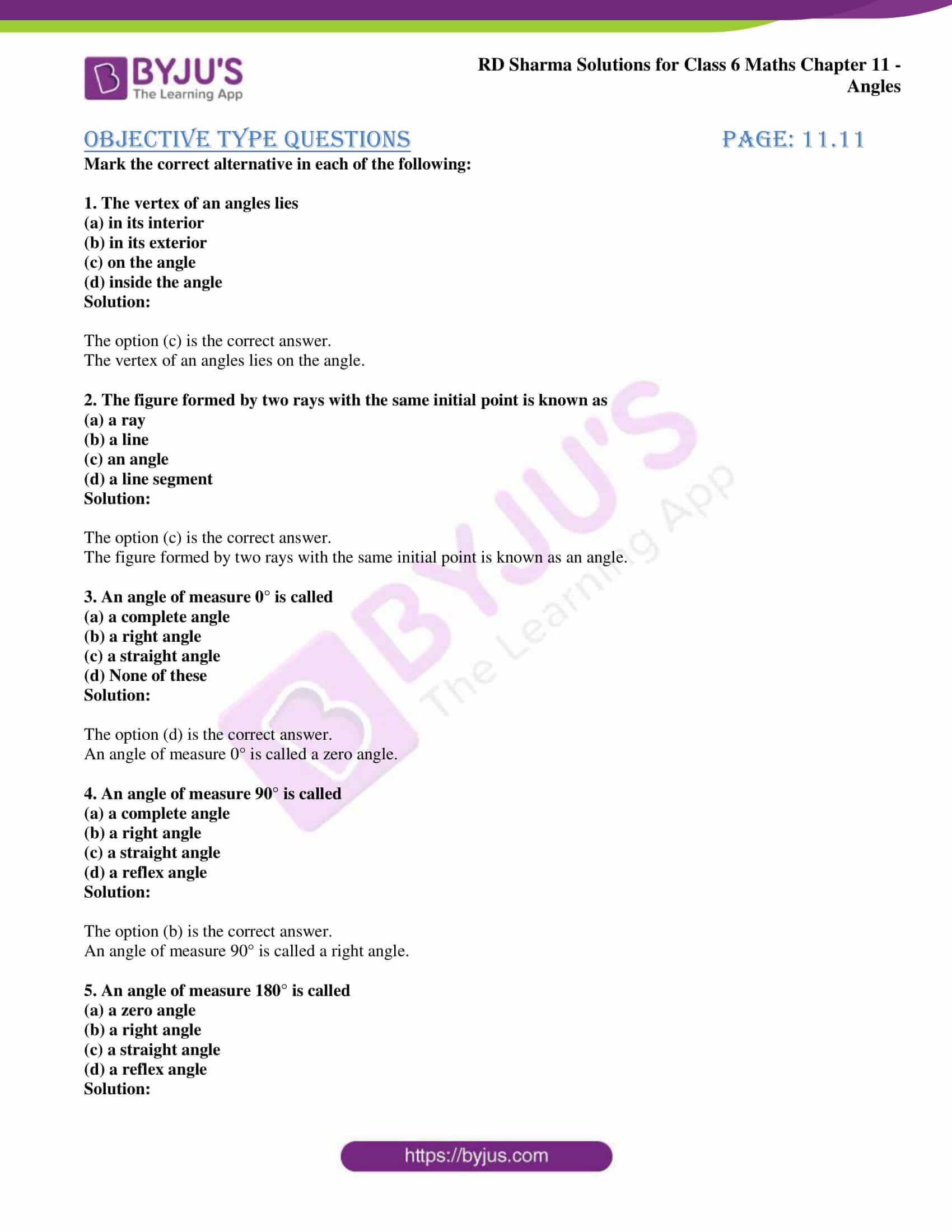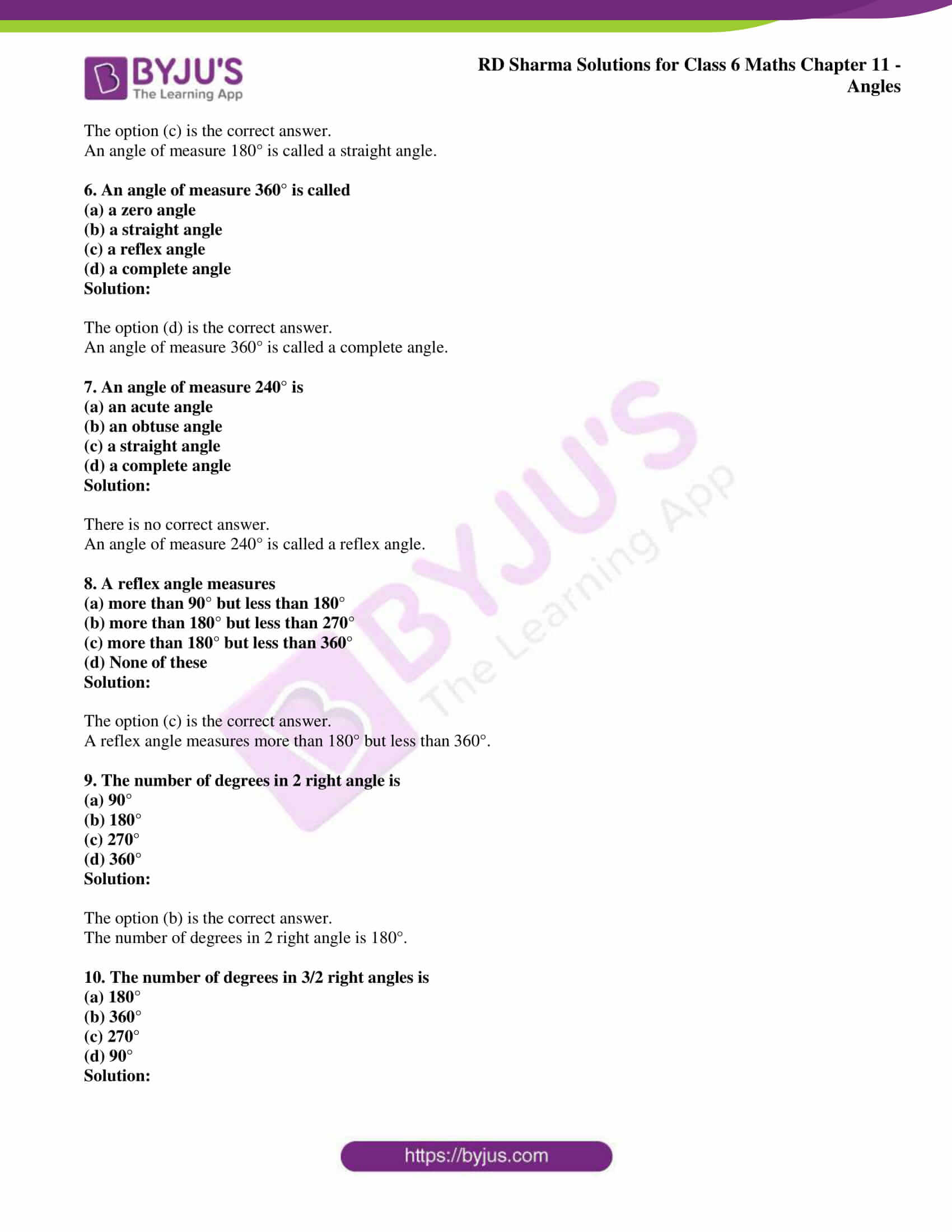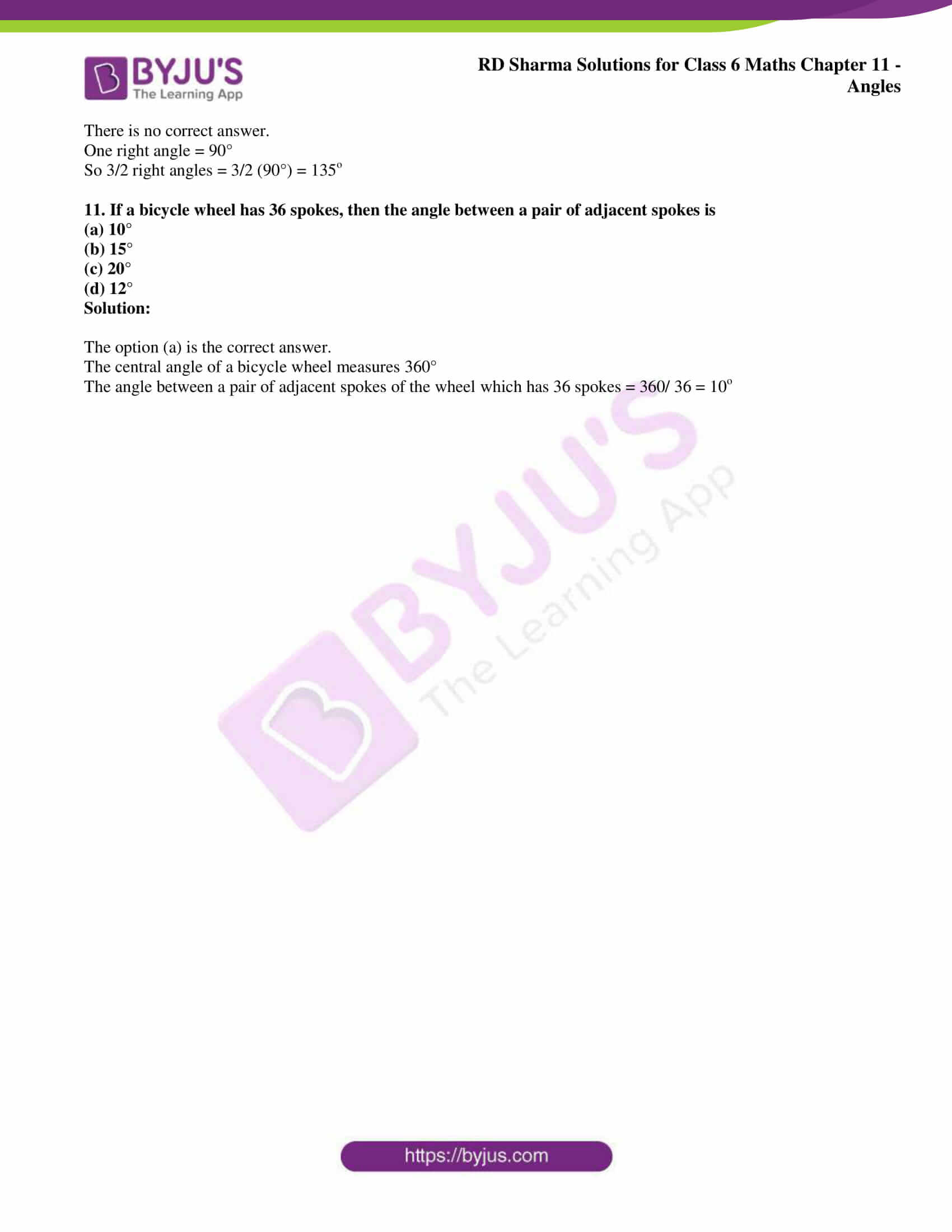# RD Sharma Solutions for Class 6 Maths Chapter 11: Angles Objective Type Questions

The objective type questions which are present at the end of this Chapter help students self analyse their knowledge about the entire Chapter. Shortcuts and tricks are explained in the solutions to help students answer the questions in a shorter duration. These solutions are prepared with the aim of assisting students to get doubts cleared by themselves and to score good marks in the exam. RD Sharma Solutions Class 6 Maths Chapter 11 Angles Objective Type Questions PDF are provided here.

## RD Sharma Solutions for Class 6 Maths Chapter 11: Angles Objective Type Questions Download PDF### Access answers to Maths RD Sharma Solutions for Class 6 Chapter 11: Angles Objective Type Questions

Mark the correct alternative in each of the following:

1. The vertex of an angles lies
(a) in its interior
(b) in its exterior
(c) on the angle
(d) inside the angle

Solution:

The option (c) is the correct answer.

The vertex of an angles lies on the angle.

2. The figure formed by two rays with the same initial point is known as
(a) a ray
(b) a line
(c) an angle
(d) a line segment

Solution:

The option (c) is the correct answer.

The figure formed by two rays with the same initial point is known as an angle.

3. An angle of measure 0° is called
(a) a complete angle
(b) a right angle
(c) a straight angle
(d) None of these

Solution:

The option (d) is the correct answer.

An angle of measure 0° is called a zero angle.

4. An angle of measure 90° is called
(a) a complete angle
(b) a right angle
(c) a straight angle
(d) a reflex angle

Solution:

The option (b) is the correct answer.

An angle of measure 90° is called a right angle.

5. An angle of measure 180° is called
(a) a zero angle
(b) a right angle
(c) a straight angle
(d) a reflex angle

Solution:

The option (c) is the correct answer.

An angle of measure 180° is called a straight angle.

6. An angle of measure 360° is called
(a) a zero angle
(b) a straight angle
(c) a reflex angle
(d) a complete angle

Solution:

The option (d) is the correct answer.

An angle of measure 360° is called a complete angle.

7. An angle of measure 240° is
(a) an acute angle
(b) an obtuse angle
(c) a straight angle
(d) a complete angle

Solution:

An angle of measure 240° is called a reflex angle.

8. A reflex angle measures
(a) more than 90° but less than 180°
(b) more than 180° but less than 270°
(c) more than 180° but less than 360°
(d) None of these

Solution:

The option (c) is the correct answer.

A reflex angle measures more than 180° but less than 360°.

9. The number of degrees in 2 right angle is
(a) 90°
(b) 180°
(c) 270°
(d) 360°

Solution:

The option (b) is the correct answer.

The number of degrees in 2 right angle is 180°.

10. The number of degrees in 3/2 right angles is
(a) 180°
(b) 360°
(c) 270°
(d) 90°

Solution:

One right angle = 90°

So 3/2 right angles = 3/2 (90°) = 135o

11. If a bicycle wheel has 36 spokes, then the angle between a pair of adjacent spokes is
(a) 10°
(b) 15°
(c) 20°
(d) 12°

Solution:

The option (a) is the correct answer.

The central angle of a bicycle wheel measures 360°

The angle between a pair of adjacent spokes of the wheel which has 36 spokes = 360/ 36 = 10o